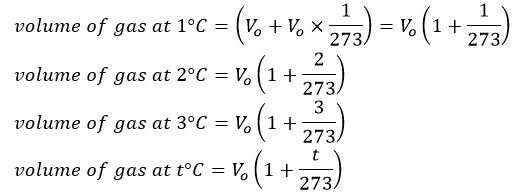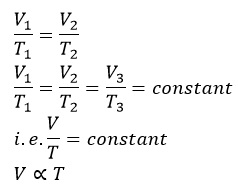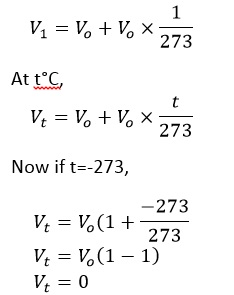# Charle’s Law

Jacquec Charles studied the variation of volume of given mass of gas with temperature at constant pressure. The result of his study are stated in the form of law which is known as Charle’s Law.
It states that, “At a constant pressure, the volume of a given mass of a gas increases or decreases by 1/273 of its volume at 0°C for each degree Celsius rise or fall in temperature.’’
Let Vo be the volume of a given mass of a gas at 0°C. Then, according to Charle’s Law.Now, if V1 be the volume of gas at t1 and V2 be the volume of gas at t­2 then

Where T = t + 273 and T is the absolute scale or kelvin scale of temperature. i.e. T1 and T2 are corresponding absolute temperature.
From the above expression,Hence, Charle’s law can also be stated as “The volume of a given mass is directly proportional to the absolute scale of temperature at constant pressure.”

Let us suppose Vo be the volume of given mass of a gas at 0°C then at 1°C,So, at -273oC the gas should occupy 0 volume theoretically. However, all the gases liquefy much more before this temperature is attend. So, this law becomes no longer valid.
Charle’s law is verified experimentally by plotting a graph between volume and temperature at constant pressure which gives a straight line. This line extrapolation intersects the temperature axis at -273oC.

From the above graph it is clear that the volume of the gas become 0 at -273oC theoretically. This is the lowest possible temperature at which the gas occupies zero volume and has no kinetic energy.